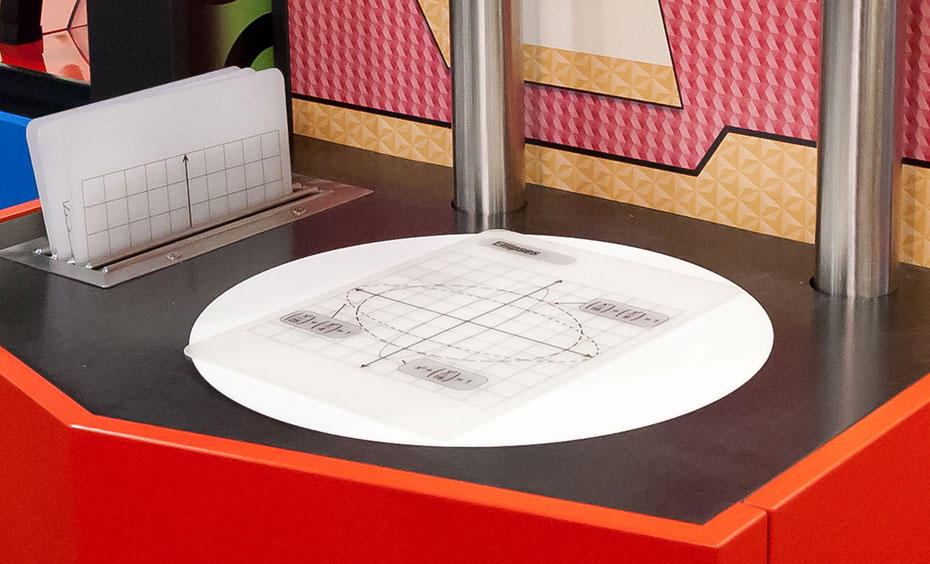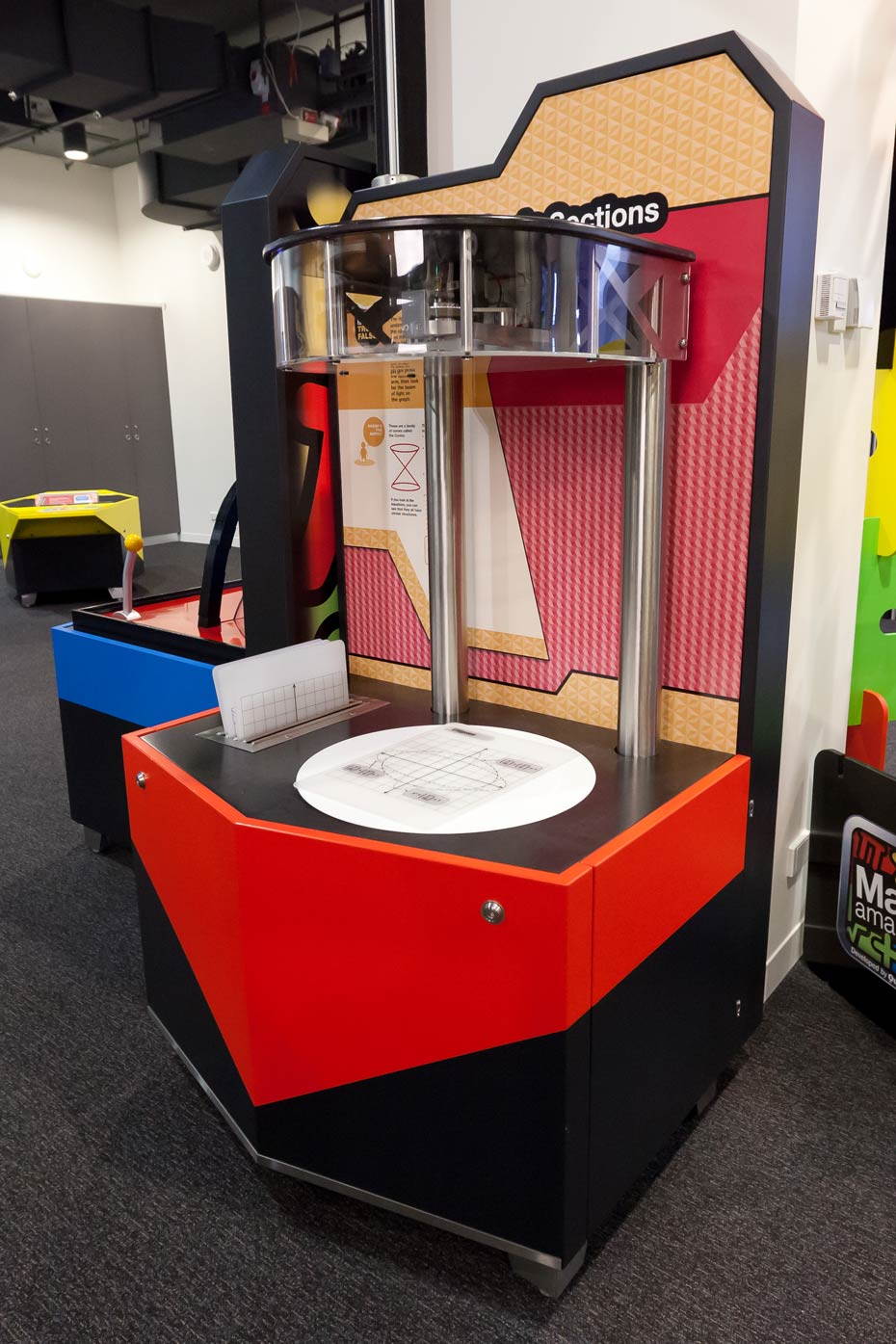# Conic SectionsMathematical curves can be found in different shapes.

## How it works

Hold various panels printed with the graph of a circle, hyperbola, parabola or ellipse over a spinning light and see how each curved shape can be found within the cone of light.

## Things to try or ask around the exhibit

• How does the laser fall across each curve?
• How are these curves all similar?
• Where might you find elliptical shapes at home?

## Background

The ‘Conics’ is a family of curves found in circles, parabolas, ellipses and hyperbolas. Parabolas describe the trajectories of objects, and are important in reflectors such as headlights. Ellipses describe how planets orbit and can be used for specialised gears. Hyperbolas, which are the only open curves in this family, describe the wake of a boat, how light diffracts, even the curves on a sharpened pencil!